Test: Basic Concept of Rotating Electrical Machines of Electrical Machines- 2

# Test: Basic Concept of Rotating Electrical Machines of Electrical Machines- 2

Test Description

## 15 Questions MCQ Test Topicwise Question Bank for Electrical Engineering | Test: Basic Concept of Rotating Electrical Machines of Electrical Machines- 2

Test: Basic Concept of Rotating Electrical Machines of Electrical Machines- 2 for Electrical Engineering (EE) 2023 is part of Topicwise Question Bank for Electrical Engineering preparation. The Test: Basic Concept of Rotating Electrical Machines of Electrical Machines- 2 questions and answers have been prepared according to the Electrical Engineering (EE) exam syllabus.The Test: Basic Concept of Rotating Electrical Machines of Electrical Machines- 2 MCQs are made for Electrical Engineering (EE) 2023 Exam. Find important definitions, questions, notes, meanings, examples, exercises, MCQs and online tests for Test: Basic Concept of Rotating Electrical Machines of Electrical Machines- 2 below.
Solutions of Test: Basic Concept of Rotating Electrical Machines of Electrical Machines- 2 questions in English are available as part of our Topicwise Question Bank for Electrical Engineering for Electrical Engineering (EE) & Test: Basic Concept of Rotating Electrical Machines of Electrical Machines- 2 solutions in Hindi for Topicwise Question Bank for Electrical Engineering course. Download more important topics, notes, lectures and mock test series for Electrical Engineering (EE) Exam by signing up for free. Attempt Test: Basic Concept of Rotating Electrical Machines of Electrical Machines- 2 | 15 questions in 45 minutes | Mock test for Electrical Engineering (EE) preparation | Free important questions MCQ to study Topicwise Question Bank for Electrical Engineering for Electrical Engineering (EE) Exam | Download free PDF with solutions
 1 Crore+ students have signed up on EduRev. Have you?
Test: Basic Concept of Rotating Electrical Machines of Electrical Machines- 2 - Question 1

### A 6-pole machine is rotating at a speed of 1200 rpm. This speed in electrical radians per sec and mechanical radian per sec are respectively given by

Detailed Solution for Test: Basic Concept of Rotating Electrical Machines of Electrical Machines- 2 - Question 1

Given,
N = 1200 rpm
∴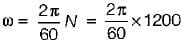We know that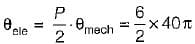Test: Basic Concept of Rotating Electrical Machines of Electrical Machines- 2 - Question 2

### In an alternator, frequency per revolution is equal to

Detailed Solution for Test: Basic Concept of Rotating Electrical Machines of Electrical Machines- 2 - Question 2

f = NP/120 cycles/sec
or,  frequency/rev = Number of pole pairs

Test: Basic Concept of Rotating Electrical Machines of Electrical Machines- 2 - Question 3

### A three - phase alternator is wound with a 60 degree phase spread armature winding and develops 300 kVA. If the armature is reconnected utilizing all the coils for single-phase operation with a phase spread of 180 degree, the new rating of the machine will be

Detailed Solution for Test: Basic Concept of Rotating Electrical Machines of Electrical Machines- 2 - Question 3

kVA rating, αEf ∝ kd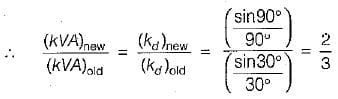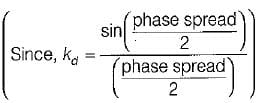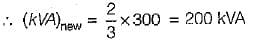Test: Basic Concept of Rotating Electrical Machines of Electrical Machines- 2 - Question 4

A synchronous machine has full-pitch coils having coil-span of 12 slots. For eliminating third harmonic, the coil-span should be

Detailed Solution for Test: Basic Concept of Rotating Electrical Machines of Electrical Machines- 2 - Question 4

To eliminate 3rd harmonic,
3α/2 = 90º
or,
α = 60°
∴ coil span = 180° - 60° = 120°
Now, 180° elec occupy 12 slots.
∴ 120° elec occupy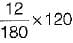= 8 slots
Hence, coil span for eliminating 3rd harmonic = 8 slots.

Test: Basic Concept of Rotating Electrical Machines of Electrical Machines- 2 - Question 5

The 5th harmonic mmf wave, produced by 3-phase currents flowing in 3-phase balanced winding, rotates w.r.t fundamental field (Ns = speed of fundamental mmf wave) at a speed of

Detailed Solution for Test: Basic Concept of Rotating Electrical Machines of Electrical Machines- 2 - Question 5

5th harmonic is a negative sequence quantity which rotates at a speed of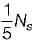w.r.t observer in space while the fundamental field rotates at a speed of Ns in space.
The direction of rotation of 5th harmonic mmf is opposite to that of fundamental mmf wave. Hence, speed of fifth harmonic w.r.t fundamental mmf wave.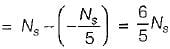Test: Basic Concept of Rotating Electrical Machines of Electrical Machines- 2 - Question 6

Match List- I (Power losses) with List - II (Dependent upon) and select the correct answer using the codes given below the lists:
List-I
B. Brush constant loss
C. Hysteresis loss
List-Il
2. Value of flux
3. Rotor rotation
Codes:
A B C D
(a) 3 1 4 2
(b) 4 1 2 3
(c) 3 4 2 1
(d) 4 1 3 2

Detailed Solution for Test: Basic Concept of Rotating Electrical Machines of Electrical Machines- 2 - Question 6

Hysteresis loss,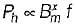Brush constant loss = VDIL  ∝ IL
Stray load loss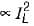No-load rotational loss (friction and windage loss) is due to rotor rotation.

Test: Basic Concept of Rotating Electrical Machines of Electrical Machines- 2 - Question 7

Output rating of
1. dc generators is in kVA.
2. ac motor is in kW.
3. ac generator is in kVA.
4. dc motors is in kW.
5. induction motors is in h.p.
6. synchronous motors is in kVA.
From these, the correct statements are:

Test: Basic Concept of Rotating Electrical Machines of Electrical Machines- 2 - Question 8

If Pi be the iron loss and P0 be the ohmic ioss on full load, then which of the following conditions has to be satisfied to obtain maximum efficiency at 2/3 full load?

Detailed Solution for Test: Basic Concept of Rotating Electrical Machines of Electrical Machines- 2 - Question 8

At maximum efficiency, copper loss = iron loss or, x2P0 - Pi (x = fraction of full load)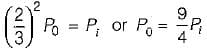Test: Basic Concept of Rotating Electrical Machines of Electrical Machines- 2 - Question 9

If ϕm is the maximum value of flux due to any one phase, the resultant flux in 3-phase and 2-phase ac machines would be given by

Detailed Solution for Test: Basic Concept of Rotating Electrical Machines of Electrical Machines- 2 - Question 9

If n be the number of phases then,
magnitude of resultant flux = n/2 ϕm
(n = 3 for 3-phase and n = 2 for 2-phase)
∴ For 3 phase,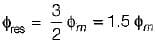and for 2 phase,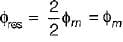Both the resultant flux will be rotating.

Test: Basic Concept of Rotating Electrical Machines of Electrical Machines- 2 - Question 10

Resistance of one conductor for a dc machine is 10 mΩ. What would be the armature circuit resistance if the dc machine with 640 conductors and 8 poles is wave-connected ?

Detailed Solution for Test: Basic Concept of Rotating Electrical Machines of Electrical Machines- 2 - Question 10

For wave-winding, number of conductors per parallel path
= Z/2 = 640/2 = 320
∴ Armature circuit resistance,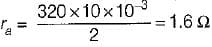Test: Basic Concept of Rotating Electrical Machines of Electrical Machines- 2 - Question 11

A 6-pole, 50 Hz, 3-phase induction motor has a stator bore diameter of 1.2 meters, its stator is cut and laid out flat, so that rotating mmf wave now becomes a travelling mmf wave. What is the linear velocity of the travelling mmf wave in meters per second ?

Detailed Solution for Test: Basic Concept of Rotating Electrical Machines of Electrical Machines- 2 - Question 11

Speed of rotating mmf wave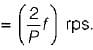As in one revolution, a peripheral distance of πD meter is traversed, therefore speed of travelling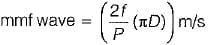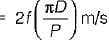= [2f (Poie-pitch)] m/s N
Now, D = 1.2 m , P = 6
∴ Speed of travelling mmf wave is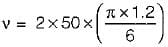= 62.83 m/s

Test: Basic Concept of Rotating Electrical Machines of Electrical Machines- 2 - Question 12

Assertion (A) : In a rotating electrical machine, having 2 poles on the stator and 4 poles on the rotor, the net electromagnetic torque developed is zero.
Reason (R) : In all rotating electrical machines, the number of rotor poles must be equal to the number of stator poles for development of electromagnetic torque.

Test: Basic Concept of Rotating Electrical Machines of Electrical Machines- 2 - Question 13

Assertion (A) : Exciting winding is one which produces working flux.
Reason (R) : Armature winding in one in which the working emf is induced by the working flux,

Test: Basic Concept of Rotating Electrical Machines of Electrical Machines- 2 - Question 14

Assertion (A) : At the time of winding design of three-phase alternators, the attention is mainly directed for the attenuation of triplen harmonics, by adopting a chording angle of 60° electrical.
Reason (R): Three-phase alternators are invariably star-connected to eliminate triplen harmonics from the line e.m.f.

Detailed Solution for Test: Basic Concept of Rotating Electrical Machines of Electrical Machines- 2 - Question 14

At the time of winding design of 3-phase alternators, the attention is mainly directed for the attenuation of non-triplen harmonics (i.e. 5th and 7th harmonics) because the triplen harmonics (3rd, 9th etc) can be automatically eliminated from line emf if alternator is connected in star.

Test: Basic Concept of Rotating Electrical Machines of Electrical Machines- 2 - Question 15

The induced emf in the armature conductors of a d.c motor is

## Topicwise Question Bank for Electrical Engineering

207 tests
 Use Code STAYHOME200 and get INR 200 additional OFF Use Coupon Code
Information about Test: Basic Concept of Rotating Electrical Machines of Electrical Machines- 2 Page
In this test you can find the Exam questions for Test: Basic Concept of Rotating Electrical Machines of Electrical Machines- 2 solved & explained in the simplest way possible. Besides giving Questions and answers for Test: Basic Concept of Rotating Electrical Machines of Electrical Machines- 2, EduRev gives you an ample number of Online tests for practice

207 tests